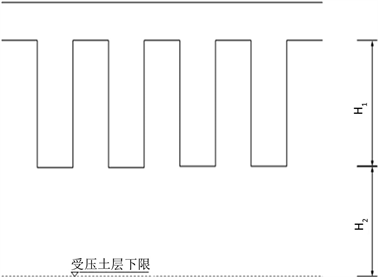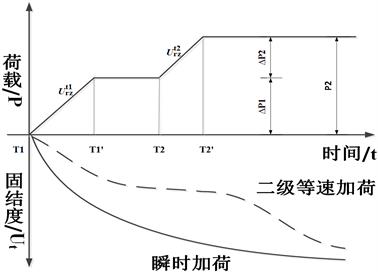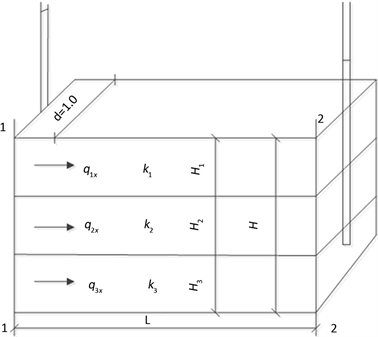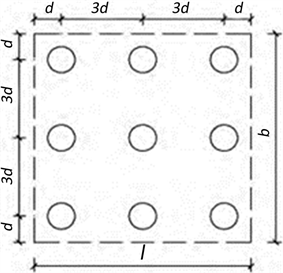﻿ 加权平均概念在岩土工程学科中的应用

加权平均概念在岩土工程学科中的应用Application of Weighted Average Concept in Geotechnical Engineering Courses

Abstract: In order to seek the general expressions for solving related physical variables in geotechnical engineering, in view of the fact that there are many diverse and complex formulas for calculating various variables in geotechnical engineering disciplines, and based on the principle of weighted average, in this paper the formulas of weighted average bulk density, average degree of consolidation and bearing capacity of composite foundation are sorted and analyzed. It is also verified through engineering cases, which has reference value for other disciplines to use the weighted average concept.

1. 前言

1) 算术平均数公式 ${A}_{n}=\frac{{a}_{1}+{a}_{2}+\cdots +{a}_{n}}{n}$

2) 加权平均数公式：若n个数为 ${a}_{1},{a}_{2},{a}_{3},\cdots ,{a}_{n}$ 的权分别是 ${w}_{1},{w}_{2},{w}_{3},\cdots ,{w}_{n}$

n = 2时，加权平均公式的简化形式为：

$\stackrel{¯}{{A}_{n}}=\frac{{w}_{1}}{{w}_{1}+{w}_{2}}{a}_{1}+\frac{{w}_{2}}{{w}_{1}+{w}_{2}}{a}_{2}=\frac{{w}_{1}}{{w}_{1}+{w}_{2}}{a}_{1}+\left(1-\frac{{w}_{1}}{{w}_{1}+{w}_{2}}\right){a}_{2}$

2. 物理指标

2.1. 饱和度

${\rho }_{sat}=n{\rho }_{w}+\left(1-n\right){\rho }_{s}$ (1)

2.2. 加权平均容重

${\gamma }_{m}=\frac{{\sum }_{i=1}^{n}{H}_{i}}{H}{\sum }_{i=1}^{n}{\gamma }_{i}$ (2a)

${\gamma }_{m}=\frac{{H}_{1}}{H}{\gamma }_{1}+\frac{{H}_{2}}{H}{\gamma }_{2}+\cdots +\frac{{H}_{n}}{H}{\gamma }_{n}$ (2b)

3. 力学指标

3.1. 复合模量

${E}_{sp}=m{E}_{p}+\left(1-m\right){E}_{s}$ (3)

3.2. 固结度

$U=\frac{{H}_{1}}{{H}_{1}+{H}_{2}}{U}_{z1}+\frac{{H}_{2}}{{H}_{1}+{H}_{2}}{U}_{z2}$ (4)Figure 1. The vertical drainage body does not penetrate the entire compressed soil layer

Uz1为竖向排水体范围内土层的平均固结度；

Uz2为竖向排水体以下部分的土层平均固结度。

3.3. 多级加载的平均固结度计算

3.3.1. 改进的太沙基法

${{U}^{\prime }}_{t}=\frac{\Delta {p}_{i}}{{\sum }_{i=1}^{n}\Delta {p}_{i}}{\sum }_{j=1}^{2}{U}_{rz}^{{t}_{j}}$ (5a)

$t>{{T}^{\prime }}_{2}$ 时：

${{U}^{\prime }}_{t}=\frac{\Delta {p}_{1}}{\Delta {p}_{1}+\Delta {p}_{2}}{U}_{rz}^{{t}_{1}}+\frac{\Delta {p}_{2}}{\Delta {p}_{1}+\Delta {p}_{2}}{U}_{rz}^{{t}_{2}}$ (5b)

${t}_{j}=t-\frac{{T}_{j}+{{T}^{\prime }}_{j}}{2}$ (6)3.4. 水平渗透的等效渗透系数

${k}_{x}=\frac{1}{H}{\sum }_{j=1}^{n}{k}_{j}{H}_{j}$ (7a)

${k}_{x}=\frac{{H}_{1}}{H}{k}_{1}+\frac{{H}_{2}}{H}{k}_{2}+\cdots +\frac{{H}_{n}}{H}{k}_{n}$ (7b)Figure 3. Schematic diagram of horizontal equivalent permeability coefficient of layered soil

3.5. 复合地基

3.5.1. 复合土体强度指标

${\tau }_{sp}=m{\tau }_{p}+\left(1-m\right){\tau }_{s}$ (8)

${C}_{sp}=m{C}_{p}+\left(1-m\right){C}_{s}$ (9)

$\mathrm{tan}{\phi }_{c}=\mathrm{tan}{\phi }_{p}+\left(1-m\right)\mathrm{tan}{\phi }_{s}$ (10)

3.5.2. 散体材料增强体复合地基承载力特征值

${f}_{spk}=m{f}_{pk}+\left(1-m\right){f}_{sk}$ (11)

3.5.3. 有黏结强度增强体复合地基承载力特征值

${f}_{spk}=\lambda m\frac{{R}_{a}}{{A}_{p}}+\beta \left(1-m\right){f}_{sk}$ (12)

3.5.4. 多型桩复合地基承载力特征值

${f}_{spk}={m}_{1}\frac{{\lambda }_{1}{R}_{a1}}{{A}_{p1}}+{m}_{2}\frac{{\lambda }_{2}{R}_{a2}}{{A}_{p2}}+\beta \left(1-{m}_{1}-{m}_{2}\right){f}_{sk}$ (13)

4. 案例

4.1. 工程概况Table 1. Comprehensive table of physical and mechanical properties of each rock and soil layer

$m=\frac{{d}^{2}}{{d}_{e}^{2}}=\frac{{d}^{2}}{{\left(1.13s\right)}^{2}}=\frac{{0.6}^{2}}{{\left(1.13×1.8\right)}^{2}}=0.087$ 代入公式(11)得：

${f}_{spk}=m{f}_{pk}+\left(1-m\right){f}_{sk}=0.087×\left[\left(\frac{1041}{\pi ×{0.3}^{2}}\right)×0.5\right]+\left(1-0.087\right)×160=306.32\text{\hspace{0.17em}}\text{kPa}$Figure 4. Schematic diagram of independent foundation pile layout (d is pile diameter)

4.2. 数据对比

5. 结论

1) 加权平均概念在土的物理性质指标及力学性质指标中有广泛的应用，通过加权平均算法，总结土的物理性质指标及力学性质指标中计算各种变量的公式，使人们更科学、直观了解加权平均容重、平均固结度、复合地基承载力等物理变量。

2) 本文通过将岩土工程学科中含有加权平均概念的公式进行归纳总结，对其他学科应用加权平均概念起到了借鉴、推广作用，有一定的参考价值。

 李广信, 张丙印, 于玉贞, 编. 土力学[M], 第2版. 北京: 清华大学出版社, 2013.

 贺建清, 雷勇, 陈伟, 编. 地基处理[M]. 第2版. 北京: 机械工业出版社, 2008.

 姚铭富. 土工试验中土的物理力学指标影响因素分析[J]. 黑龙江水科技, 2018, 46(11): 110-111+114.

 王丽, 林彤. 堆载压法处理软土地基的固结度分析[J]. 山西建筑, 2006, 32(3): 101-102.

 张庚成, 赵洪辉, 王新强. 大型储罐复合地基承载力计算分析[J]. 中国水运(下半月), 2020, 20(05):251-252.

 陈立华, 李维斌, 吴伟杰. 复合地基沉降计算复合土层压缩模量取值的探讨[J]. 水泥工程, 2019(4): 89-90.

 曲长武, 胥新伟. 刚性桩复合地基承载力特性试验研究[J]. 中国港湾建设, 2020, 40(4): 41-45.

 马良. 高层建筑CFG桩复合地基设计研究[J]. 价值工程, 2020, 39(9): 145-146.

 李雅婷, 毛卉, 李森林. 强夯碎石桩复合地基承载力的计算方法简析[J]. 港工技术, 2019, 56(S1): 138-141.

 闫明礼, 曲秀莉, 刘伟, 孟宪忠, 闫雪晖. 复合地基的复合模量分析[J]. 建筑科学, 2004, 20(4): 27-32.

 闫明礼, 罗鹏飞, 佟建兴, 孙训海. 复合地基面积置换率[J]. 工程勘察, 2014, 42(9): 30-35.

Top